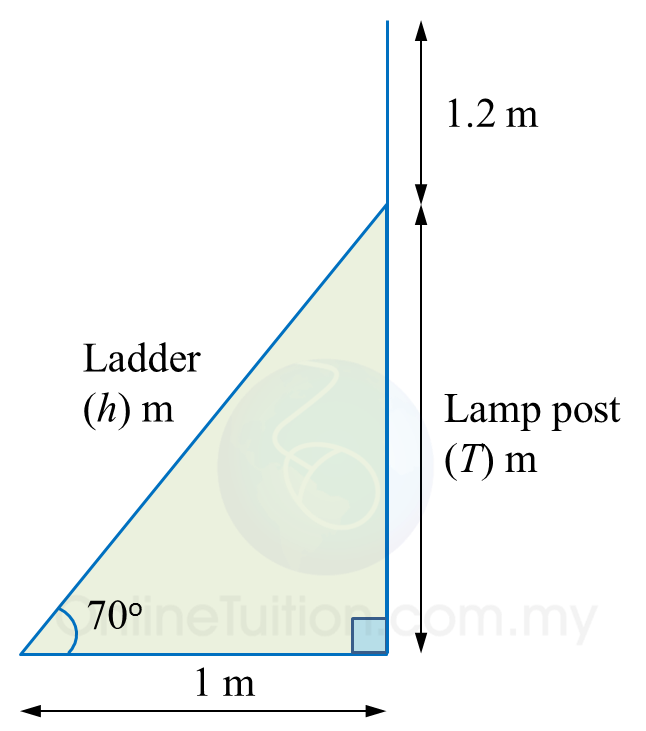# 5.2.4 Trigonometric Ratios, PT3 Focus Practice

Question 9:
Diagram below shows a vertical pole, PQ. At 2.30 p.m. and 5.00 p.m., the shadow of the pole falls on QR and QS respectively.Calculate
(a) the height, in m, of the pole.
(b) the value of w.

Solution:
(a)

(b)

Question 10:
A technician needs to climb a ladder to repair a street lamp as shown in Diagram below.(a) What is the length, in m, of the ladder?
(b) Find the height, in m, of the lamp post.

Solution:(a)

(b)